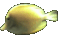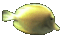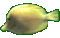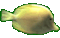Graphs of Lines in Slope Point Form     Math Help     Game Tips:

- Each of the three lines represented by y=2x, y=3x, and y=4x passes through the point P(0,0).
In general, a line 'y=mx' has slope 'm' and passes through the point (0,0).

- The two lines represented by (y-5)=2(x-1) and (y-5)=3(x-1) will each pass through the point P(1,5).
In general, a line y-y1=m(x-x1) has slope m and passes through the point P(x1,y1).

- When comparing the line y=2x to the line y-5=2(x-1), the two parallel lines have the same slope=2
and y-5=2(x-1) is shifted one unit East and five units North compared to y=2x.
The line y-5=2(x-1) passes through the point P(1,5).

- When comparing the line y=6x to the line y+7=6(x-4), the two parallel lines have the same slope=6
and y+7=6(x-4) is translated 4 units in the positive x direction and 7 units in the negative y direction.
The line y+7=6(x-4) passes through the point P(4,-7).

- When point P(x1,y1) is on the y-axis, it can be represented as the point P(0,b)
and the line y-y1=m(x-x1) becomes y-b=m(x-0) , which is equivalent to y=mx+b.

- When point P(x1,y1) is on the x-axis, it can be represented as the point P(a,0)
and the line y-y1=m(x-x1) becomes y-0=m(x-a) , which is equivalent to y=m(x-a).

- Your Game Score is reduced by the number of fish hits.

- To slow the game speed repeat tap/click on the word Slider.
- To increase the game speed repeat tap/click on the word Math.
- Speed can also be adjusted with a keyboard's - and + keys.

- Refresh/Reload the web page to restart the game.